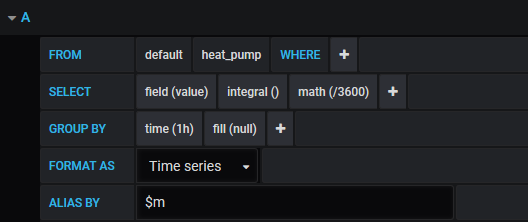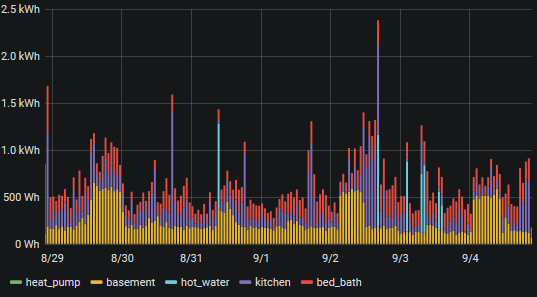# Calculating kWh

Hi guys
I recently purchased my first IOTAWATT unit and simply loving it… one question I have is to how to get kWh plotted in Grafana.
I upload data every 10 seconds and now need to calculate kWh per each circuit. By the way when I use the built in kWh in the Influxdb webpage setup section and the results don’t look right.
Any help is greatly appreciated.
Thanks

Haven’t done anything with grafana for about a year except look at my graphs, but I’ll try to steer you in the right direction. There are two ways to approach this.

The first is to simply integrate the power series. Power is Watts. So if you integrate it over the grouping, you will get Ws (Watt seconds, assuming you have your epoch set to seconds, and why wouldn’t you?). You can then divide that by 3600 to get Wh. When you specify the plot units to be Wh, grafana will automatically convert to kWh when it exceeds 999. A query looks like this:You can see that the “value” field of the heat_pump measurement is integrated over each group of 1h. The result is then divided by the number of seconds in an hour to get Wh. There are queries for a number of measurements in this panel. The result is stacked and looks like this:The second way to approach the problem is to upload Wh to influx. You can then simply use the sum() function to get the Wh for each grouping. As in the above example, grafana know about Wh and kWh and will label appropriately.

1 Like

Thank you for the help!